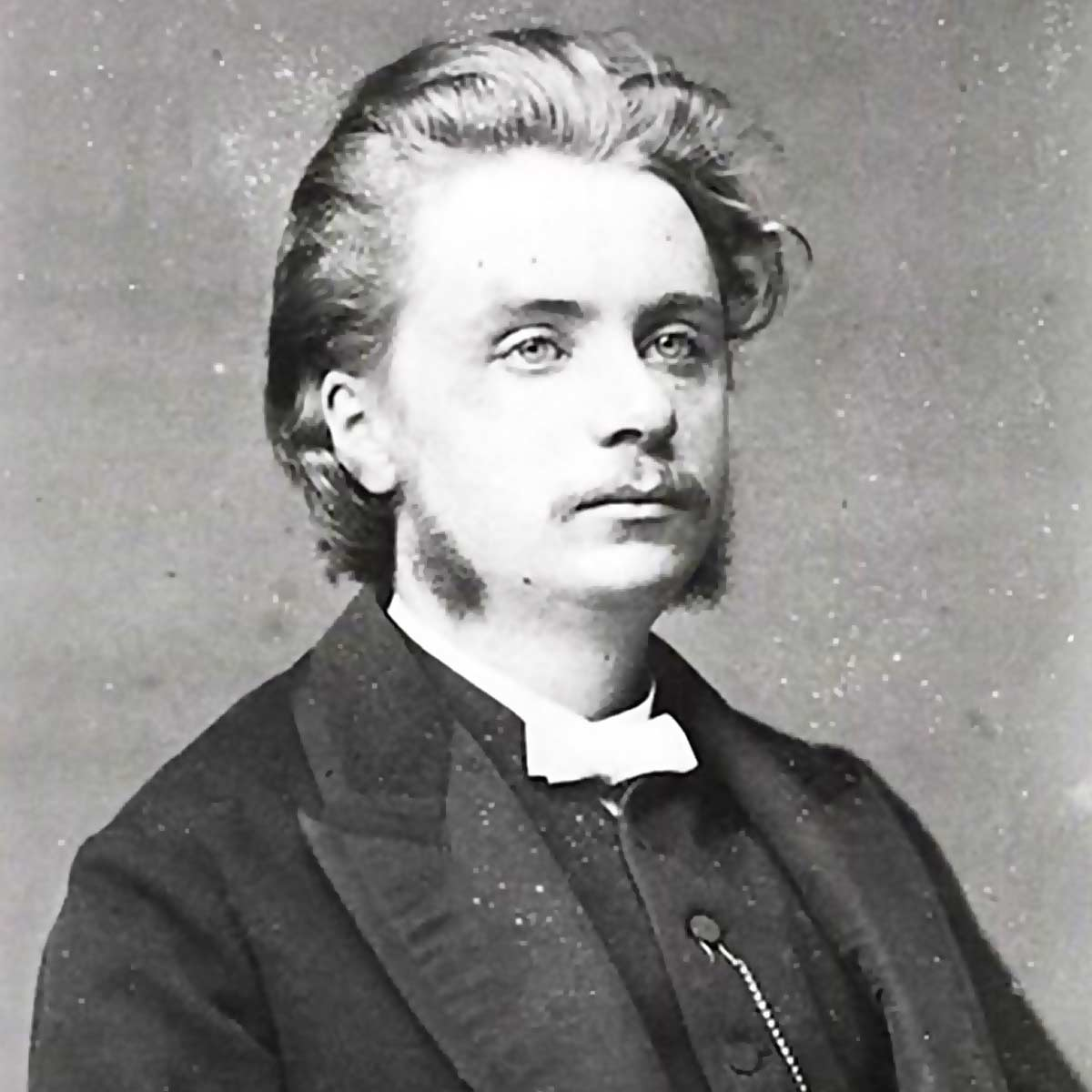# Edvard Grieg

4 Music Sheets

• ###### Birth Name:

Edvard Hagerup Grieg

• ###### Born:

15th June 1843

• ###### Genres:

Edvard Hagerup Grieg was a Norwegian composer and pianist. He is widely considered one of the leading Romantic era composers, and his music is part of the standard classical repertoire worldwide. His use and development of Norwegian folk music in his own compositions brought the music of Norway to international consciousness, as well as helping to develop a national identity, much as Jean Sibelius did in Finland and Bedrich Smetana did in Bohemia.Credit: Wikipedia

## Artist's Music Sheets

•[hE(] Y [oh] P [hE] Y [oh] P [rh] y [fO] a [rg] y O a [rg(] y [gO] a [rg] y [gO] a [gE] Y [od] P [ED] Y o P [wD8] e [YD] o [wd^] E [dY] o [ws6] t [sY] o [wP9] E Y o [wD8] e [YD] o [wd^] E [YP] [od] [ws6] t [sY] o [wP9] E Y o [wp8] e [YP] [so] d g|D [teI(]| [woE]| [i(%] q [to] [YO] a d|s [e^YQ]|| [wYE] o [h@] Y [oh] P [hE] t [oh] P [rh] y [fO] a [rg] y O a [rg(] y [gO] a [rg] y [gO] a [gE] Y [od] P [ED] Y o P [^P] 8 [PE] Y [p8] ( [ie] [pY] [O4] ( [WO] Y [o5] ( E Y [i%] ( [to] [YO] a d|s [yqW7]| [wY8(]| [i(%] q [to] [YO] P d|s [e^YQ]|| [wYE] o [h@] Y [oh] P [hE] Y [oh] P
Level: 5
Length: 00:51
Intermediate

#### Edvard Grieg

•[a 7 9 Q S] d f [QG7] d G|[qg*] S g|[f80] s f| [a 7 9 Q S] d f [QG7] d G k [j9] G [ed] G [j9]|e| [a 7 9 Q S] d f [QG7] d G|[qg*] S g|[f80] s f| [a 7 9 Q S] d f [QG7] d G k [j9] G [ed] S [a7]|| 7 * [9\$] 0 [Q7] 9 [Q\$]|[q7] * [q\$]| 8 [0\$]| 7 * [9\$] 0 [Q7] 9 [Q\$] r [e2] Q  Q [e2]|6| 7 * [9\$] 0 [Q7] 9 [Q\$]|[q7] * [q\$]| 8 [0\$]| 7 * [9\$] 0 [Q7] 9 [Q\$] r [e2] Q  Q [e2]|6| [Q\$] W [E*] r [T\$] E [T*]|[y2] E [y9]|[T\$] E [T*]| [Q\$] W [E*] r [T\$] E [T*]|[y2] E [y^]|[T\$]|*| [Q\$] W [E*] r [T\$] E [T*]|[y2] E [y9]|[T\$] E [T*]| [Q\$] W [E*] r [T\$] E [T*]|[y2] E [y^]|[T\$]|*| [r7] T [y\$] u [I7] y [I\$]|[i7] T [i\$]|[u7] t [u\$]| [r7] T [y\$] u [I7] y [I\$] a [p2] I [y6] I [p2]|6| [r7] T [y\$] u [I7] y [I\$]|[i7] T [i\$]|[u7] t [u\$]| [r5] T [y7] u [I\$] y [I7] a [P\$] I [P*] S [a7]|[yaI7]
Level: 5
Length: 00:51
Intermediate

#### Edvard Grieg

•[uth] f d s d f [uth] f d s d f [uth] f h [tje] f j [uth] f d s|| [o80] u y t y u [o80] u y t y u [o80] u o [pe] u p [aW0] O I u|| [aW0] O I u I O [aW0] O I u I O [aW0] O a [s8] O s [aW0] O I u|| [aW0] O I u I O [aW0] O I u I O [aW0] O a [se] p s [wrd] a p o|| [wrd] a p o p a [wrd] a p o p a [wrd] a o y r w d a o [wri]|| [uth] f d s d f [uth] f d s d f [uth] f h [tje] f j [yji] g f d|| [qp9] i u y u i [qp9] i u y u i [p9] i p [wa] i a [a0] o a [s8] o s [sq] p s [d9] p d [f0] W r 0 W r [qd] e t [sq] e t [a0] W r 0 W r [wa] r y w|| [o80] u y t y u [o80] u y t y u [o80] u o [pe] u p [o80] y t|| [h8] f d [se] d f [h8] f d [se] d f [h8] f d [se] d f [sh80]
Level: 6
Length: 01:38
Intermediate

#### Edvard Grieg

•[f3]||| [e6] r [t3] y [u6] t [u3]| [Y6] r [Y3]|[y6] E [y3]| [e6] r [t3] y [u6] t [u3] p [o1] u [t5] u [o1]|5| [e6] r [t3] y [u6] t [u3]| [Y6] r [Y3]|[y6] E [y3]| [e6] r [t3] y [u6] t [u3] p [o1] u [t5] u [o1]|5| [u3] I [O7] p [a3] O [a7]| [s1] O [s%]|[a3] O [a7]| [u3] I [O7] p [a3] O [a7]| [s1] O [s%]|[a3]|7| [u3] I [O7] p [a3] O [a7]| [s1] O [s%]|[a3] O [a7]| [u3] I [O7] p [a3] O [a7]| [s1] O [s%]|[a3]|7| [p6] a [s3] d [f6] s [f3]| [D6] a [D3]|[d6] P [d3]| [p6] a [s3] d [f6] s [f3] j [h1] f [s5] f [h1]|5| [p6] a [s3] d [f6] s [f3]| [D6] a [D3]|[d6] P [d3]| [p6] a [s6] d [f5] s [f4] j [f3] s [f2] j [p1]|7
Level: 4
Length: 02:16
Easy